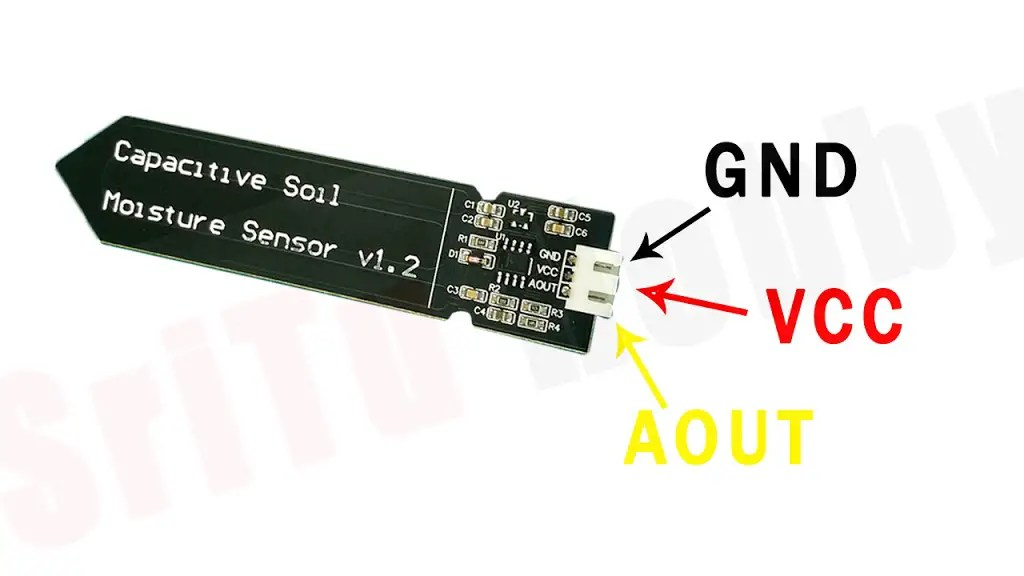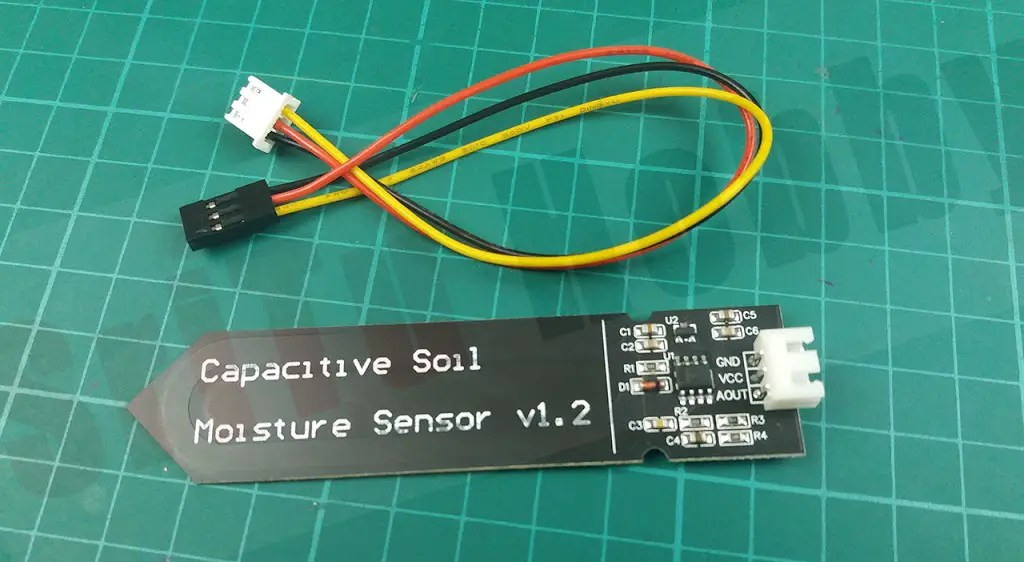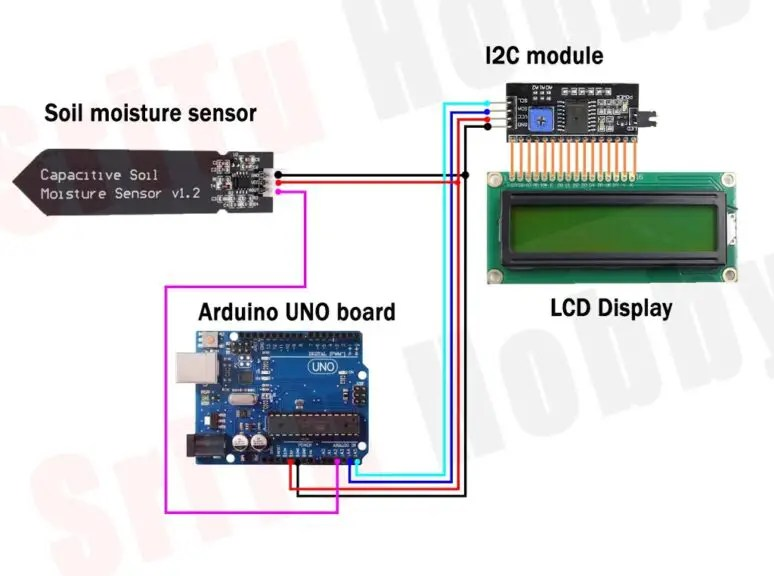# Capacitive soil moisture sensor v1.2 Arduino code | Step by step instructions

## Capacitive soil moisture sensor v1.2 Arduino codeHello guys, welcome back. Today we are going to talk about how the “capacitive soil moisture sensor ” works with Arduino. Also, soil moisture can be easily monitored by this sensor. An LCD display is also used to monitor this value. Once this sensor is inserted into the soil we can get the values ​​through this. It can be used primarily to make irrigation systems.

### How the Capacitive Soil Moisture Sensor works.Soil moisture was measured by this sensor by measuring the change in capacitance caused by changes in the electrolyte content of the soil. It also includes a 555 IC circuit to measure this capacity. This circuit produces a voltage proportional to the capacitance of the sensor. This voltage level is between 1.2v and 3v. Also, we can get that voltage through the analog pin. It is also known as soil moisture. This sensor requires a voltage of 3.2v to 5v to operate. However, the built-in voltage regulator completes the functionality and the sensor is made of corrosion-resistant material so it can be used for a long time.
We can see three pins in this sensor. Connect the cathode / GND terminal to the GND pin. A 5v voltage should be provided for the VCC terminal. Also, the AOUT PIN can obtain soil moisture as an analog value.Well, now we will learn step by step how this sensor works with Arduino. Below are the necessary components.

### Step 1

Firstly, identify these components.

#### Arduino UNO board.#### Capacitive soil moisture sensor.#### Jumper wires.### Step 2

Secondly, connect these components. For that, using the circuit diagram below.### Step 3

Thirdly, let us prepare the required program for this. It is given below.
The complete program of this project – Download
``````#include <LiquidCrystal_I2C.h>
LiquidCrystal_I2C lcd (0x27, 16, 2);
#define sensor A2
#define wet 210
#define dry 510

void setup() {
Serial.begin(9600);
lcd.init();
lcd.backlight();
}

void loop() {
int value = analogRead(sensor);
Serial.println(value);
lcd.setCursor(1, 0);
lcd.print("Moisture Value");
lcd.setCursor(3, 1);
lcd.print(value);
lcd.print(" ");
lcd.setCursor(7,1);
lcd.print("--");
int pre = map(value, wet, dry, 100, 0);
lcd.setCursor(10, 1);
lcd.print(pre);
lcd.print("%");
lcd.print(" ");
}``````

#### Code explanation

Firstly, enter the I2C library. After, an object was created for the I2C library. It then includes the I2C library address and the LCD height and width.
#include <LiquidCrystal_I2C.h>
LiquidCrystal_I2C lcd (0x27, 16, 2);

Secondly, defines the objects for sensor PIN, wet value, and dry value.
#define sensor A2
#define wet 210
#define dry 510

Thirdly, the I2c library is initialized in the void setup.
void setup() {
Serial.begin(9600);
lcd.init();
lcd.backlight();
}

Finally, values are obtained from the soil moisture sensor and added to the variable called “value”. After, the value was printed on the LCD.
void loop() {
int value = analogRead(sensor);
Serial.println(value);
lcd.setCursor(1, 0);
lcd.print(“Moisture Value”);
lcd.setCursor(3, 1);
lcd.print(value);
lcd.print(” “);
lcd.setCursor(7,1);
lcd.print(“–“);
int pre = map(value, wet, dry, 100, 0);
lcd.setCursor(10, 1);
lcd.print(pre);
lcd.print(“%”);
lcd.print(” “);
}

### Step 4

OK, now select the correct board and port. Then, upload this code.### Step 4

Now we can test this project. For that, insert the sensor into the soil bowl and view the readings on the LCD. The full video guide is given below. So, we will meet in the next tutorial.